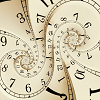#### You may also like### Decimal Time Poster

Poster based on Decimal Time task.### Online Poster### Seven Flipped Poster

Poster based on Seven Flipped task.

# Clock Face Poster

##### Age 7 to 11Challenge Level

For the numbers on both sides of the line to have the same total, the line needs to go from between the 9 and 10, to between the 3 and 4.

To divide the clock face so that the total of the numbers on one side of the lines is twice the total on the other side, the lines can go from between 10 and 11 to the centre and from between 2 and 3 to the centre; or from between 4 and 5 to the centre and from between 8 and 9 to the centre.

There are eight different ways of diving up the clock face to give two parts where the sums are prime numbers:

• With the hands going from between 4 and 5 to the centre and from between 5 and 6 to the centre
• With the hands going from between 1 and 2 to the centre and from between 3 and 4 to the centre
• With the hands going from between 6 and 7 to the centre and from between 7 and 8 to the centre
• With the hands going from between 2 and 3 to the centre and from between 4 and 5 to the centre
• With the hands going from between 10 and 11 to the centre and from between 11 and 12 to the centre
• With the hands going from between 4 and 5 to the centre and from between 6 and 7 to the centre
• With the hands going from between 7 and 8 to the centre and from between 9 and 10 to the centre
• With the hands going from between 8 and 9 to the centre and from between 10 and 11 to the centre

To see more detailed methods, please look at the published children's solutions to the original problem.